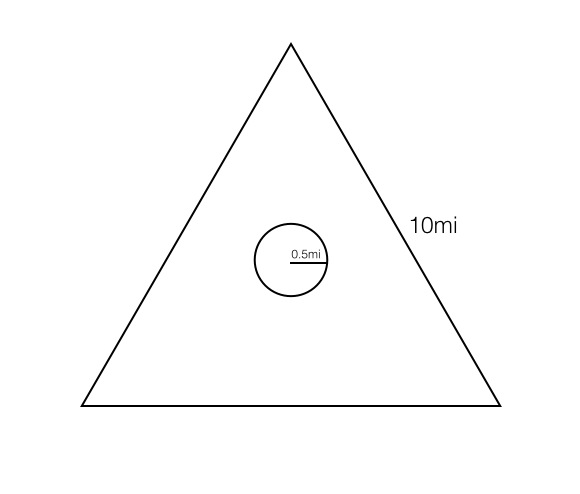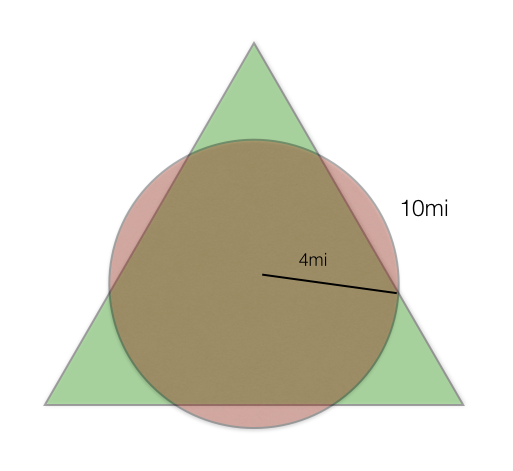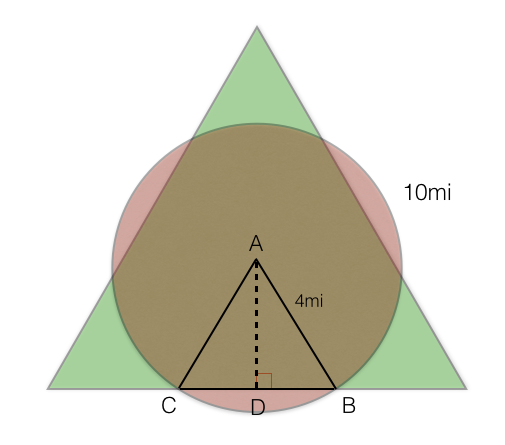## The Island Bomb Problem

2016 Nov 20

Suppose, in the middle of an ocean, there lies an island in the shape of an equilateral triangle with a side length of $10$ miles. A tiny village sits in the circumcenter of the island. The village is in the shape of a circle with a radius of $0.5$ miles. A plane carrying a bomb flies over the island and drops it at a random point on the island. The bomb destroys anything within $3.5$ miles of wherever it lands. What is the probability that part or all of the village is destroyed?

Here is a diagram illustrating the problem:Since the radius of the village is $0.5$ miles and the blast radius of the bomb is $3.5$ miles, if the bomb lands within $4$ miles of the center of the village, the villagge will be damaged, so we can update our diagram to look like this:The probability that it will damage the village is equal to the area of the overlap between the circle and the triangle (the places at which it could land on the island that would damage the village) divided by the area of the triangle (the area in which it might land). The area of the triangle is easily found by our regular polygon area formula:

Now comes the tricky part: finding the area of the overlap. By drawing line segments from the center of the circle to two of the points at which it intersects the triangle, we construct an isoscles triangle. The altitude length of this small isoscles triangle is equal to the apothem of the island, which is $\frac{5\sqrt{3}}{3}$. We can now draw the following diagram:The triangle $\triangle ACD$ is a right triangle with $\overline{AD}=\frac{5\sqrt{3}}{3}$ and $\overline{AC}=4$. By using the pythagorean theorem, we find that

And by the formula for the area of a triangle, the area of one of the right triangles is

And the area of one of the isoscles triangles formed by two of the right triangle is

There are three isoscles triangles that are formed this way, because the circle intersects the island triangle in six points, and they are all congruent, so the area of all three triangles together is

Now the area that remains for use to find is the area of the three congruent sectors of the circle in between each of the pairs of isoscles triangles. In order to find their areas, we must find the angles of each of the sectors, and in order to do this, we must find the sum of the angles opposite the bases of each of the isocles triangles (i.e., $\angle BAC$). We can use triangle trigonometry to do this by noticing that

Now we can use the sector area formula to find the area of all three of the sectors:

Then the total shaded area is the area of the three sectors together plus the area of the three triangles together:

Then the spatial probability is

So there is about an $86.71\%$ chance that the village will be damaged.